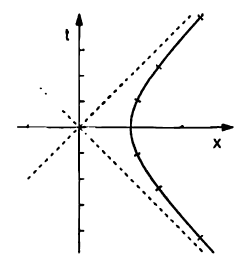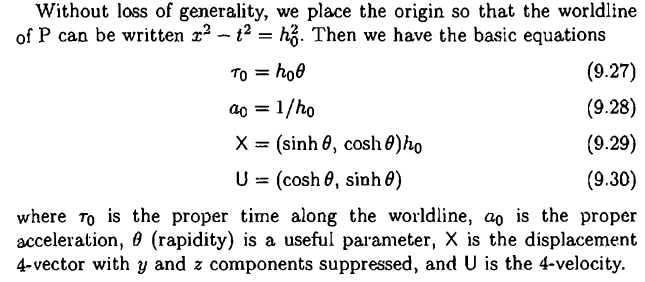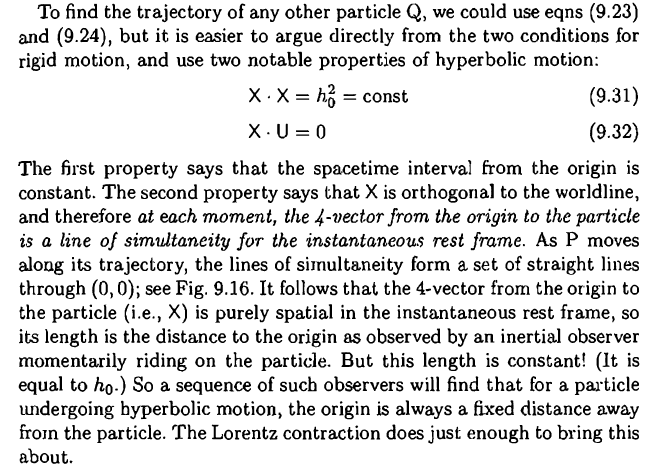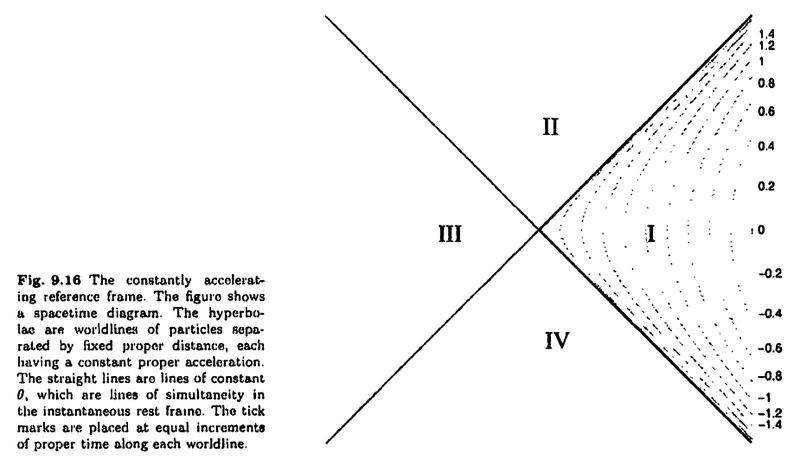# Rigidly Constantly Accelerating Frame

• unscientific

#### unscientific

We know that when a rigid frame, say a rocket undergoes constant proper acceleration, its worldline is hyperbolic. The equation is given by:

$$x^2 - c^2t^2 = \left( \frac{c^2}{a_0} \right)^2$$Suppose P is such a worldline and worldine can also be written as:I understand how these are derived up to this point. But it is the reasoning presented below that confuses me.

Things I don't understand:

• Why does ## X \cdot U = 0 ## imply that "4-vector from origin to particle is a line of simultaneity for the instantaneous rest frame" ?
• Why do these lines of simultaneity pass through (0,0)? Shouldn't they simply be ##x = \pm ct## based on the first picture?For completeness, Fig. 9.16 showing the worldlines and lines of simultaneity are shown below:In the instantaneous rest frame, ##U## is pointing in the time direction, i.e., its components are (1,0,0,0). Anything orthogonal to this has time component zero and thus a line from the origin has zero change of dt in the instantaneous rest frame, i.e., it is a surface of simultaneity in that frame.

In the instantaneous rest frame, ##U## is pointing in the time direction, i.e., its components are (1,0,0,0). Anything orthogonal to this has time component zero and thus a line from the origin has zero change of dt in the instantaneous rest frame, i.e., it is a surface of simultaneity in that frame.
That makes sense. What about point 2? I thought based on the formula of the hyperbola these lines of simultaneity should simply be ##x = \pm ct##?

##x = \pm ct## is not a surface of simultaneity in any frame. A surface of simultaneity is simply a set of events with the same time coordinate in a given inertial frame, which means they are by definition space-like. The light cone is by definition ... well ... light-like. :)

##x = \pm ct## is not a surface of simultaneity in any frame. A surface of simultaneity is simply a set of events with the same time coordinate in a given inertial frame, which means they are by definition space-like. The light cone is by definition ... well ... light-like. :)
So in fig 9.16, is there only one surface of simultaneity for different values of ##a_0##? How do I find an expression for that surface?

So in fig 9.16, is there only one surface of simultaneity for different values of ##a_0##? How do I find an expression for that surface?
No surfaces of simultaneity are drawn in 9.16 (at least not visible in that image, the original might have shown it clearer - by the way, you should also cite the original when quoting images and text). You have the light cone related to the origin and different hyperbola corresponding to different world lines with different constant proper acceleration. However, for each instantaneous rest frame for each of the world lines, they are all moving with velocity zero at the same time, i.e., time t=0 in that frame and they are equidistant at that time as well.

In addition, the light-like world line separating I and II is the Rindler horizon - no information can reach any of the observers from events contained in II or III.